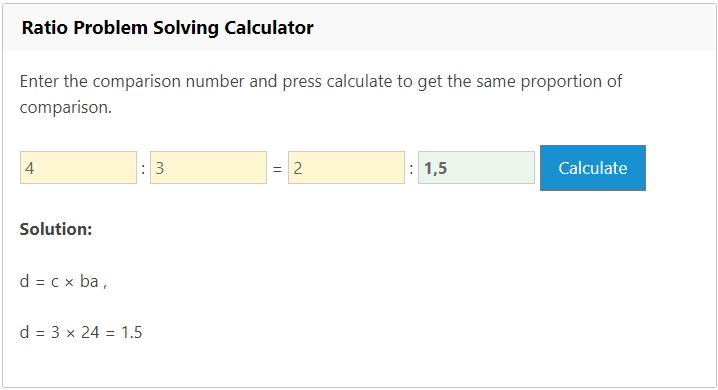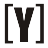# Ratio Calculator

How to Use: Insert numbers in Ratio box and automatically will calculate the result of equivalent ratios in the table below.
Ratio Calculator

:

### Ratio Calculation Results

Ratio Problem Solving Calculator

Enter the comparison number and press calculate to get the same proportion of comparison.

: = :

What is Ratio Calculator?
A ratio calculator is a tool that can be used to determine the proportion of two or more numbers. It can be used to calculate the ratio of any two quantities, Example such as the ratio of width to height of an image, or the ratio of water to flour in a recipe.
What is Ratio Problem Solving Calculator?
A ratio problem solving calculator is a tool that can be used to solve ratio problems. It can be used to calculate the missing value in a ratio problem, given the other values. For example, if the ratio of men to women in a room is 2:3 and there are 12 men, the calculator can be used to find the number of women in the room.
TIPS If you want a smaller ratio or other numbers than the results in the Equivalent Ratios Table, you can use the Ratio Problem Solving Calculator by inputting one value to get the same proportion.

Example:
Q. 4 : 3 = 2 : ?
A. 4 : 3 = 2 : 1,5### 2 thoughts on “Ratio Calculator”

1.make it so it does smaller numbers too

2.It’s not the best and it doesn’t help me at all. I don’t understand this a single bit! Maybe you can improve it by giving not only bigger numbers but also smaller ones as well? And maybe put what are equivalent to the numbers? It’ll help a lot! Please take this into thought and have a good day.YoosFuhl.com Would you like to receive notifications on latest updates? No Yes